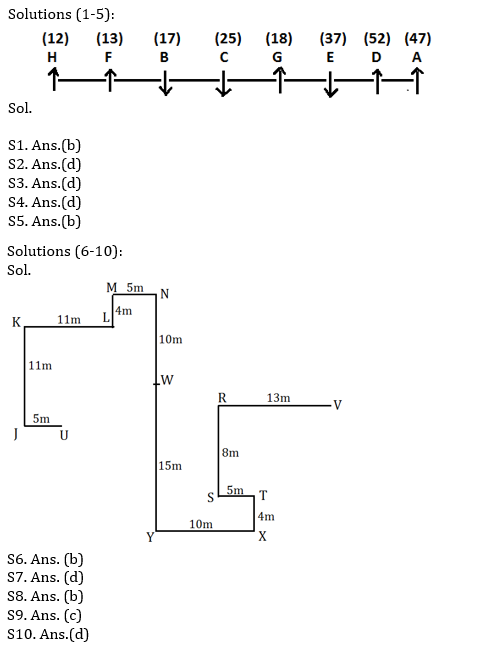Latest Banking jobs   »   Reasoning Ability Quiz For RRB PO...

# Reasoning Ability Quiz For RRB PO Mains 2021- 29th August

Directions (1-5): Study the following information carefully and answer the questions given below:
Eight persons, A, B, C, D, E, F, G, and H are sitting in a straight line (but not necessarily in the same order). Three of them are facing south and rests are facing north direction. They all have their lucky numbers i.e. 12, 13, 37, 47, 52, 17, 25, and 18 (not necessarily in same order).
F sits right of C. E’s lucky number is a prime number. G and E face opposite direction. C’s lucky number is average of E’s lucky number and F’s lucky number. B sits third from the extreme end of the row. H’s lucky number is at least less than two numbers among all the given numbers. C doesn’t face north direction. G is an immediate neighbor of C and E both. B faces south direction. B’s lucky number is less than G’s lucky number but greater than H’s lucky number. D sits 2nd to the right of G. C sits immediate left of B. H sits 3rd right of C. The one, who sit at one of the extreme ends, has lucky number is a prime number. Both persons who sits at extreme ends face same direction. All the persons who sit left of D, those lucky numbers are less than the lucky number of D who face north. E’s lucky number is greater than at least three numbers among all the given numbers. The one whose lucky number is 17 doesn’t sit, immediate right of D and immediate left of F. H sits left of F. E’s lucky number is not second highest. A’s lucky number is a prime number.

Q1. Who among the following is second to the left of the one whose lucky number is 52?
(a) The one whose lucky number is 13
(b) Both (C) and (E)
(c) G
(d) None of these
(e) The one whose lucky number is 18
L1Difficulty 3
QTags Linear seating arrangement

Q2. What is the lucky number of C?
(a) 12
(b) 47
(c) 37
(d) 25
(e) None of these

Q3. Who among the following sits third to the right of the one whose lucky number is 25?
(a) B
(b) C
(c) A
(d)H
(e) None of these

Q4. What is the position of F with respect to the one whose lucky number is 37?
(a) Second to the left
(b) Third to the left
(c) Second to the right
(d) Fourth to the right
(e) None of these

Q5. C faces in which direction?
(a) North
(b) South
(c) Same as D faces
(d) Both (a) and (c)
(e) Cannot be determined

Directions (6-10): Study the following information carefully and answer the questions given below:
P@Q: It means that P is in East of Q
P#Q: It means that P is in West of Q
P\$Q: It means that P is in North of Q
P%Q: It means that P is in South of Q
P(7)\$Q means that P is 7m North of Q
P\$@Q means that P is in North-east of Q
Shubham and Akash are starting their journey from point V and U respectively and both reaches at point W as per the given information-
Shubham- R(13)#V, S(8)%R, T(5)@S, X(4)%T, Y(10)#X, W(15)\$Y
Akash- J(5)#U, K(11)\$J, L(11)@K, M(4)\$L, N(5)@M, W(10)%N

Q6. If point A(2)%R then, what is the direction and shortest distance of A with respect to U?
(a) A(15)\$@U
(b) A(16)@U
(c) A(16)\$@U
(d) A(15)@U
(e) None of these

Q7. Which of the following is true?
(a) M\$@X
(b) U%@N
(c) U#R
(d) J%#V
(e) None is true

Q8. If B(5)@L and C(8)#W are true then, what is the shortest distance between B and C?
(a) 5m
(b) 10m
(c) 15m
(d) 20m
(e) None of these

Q9. U is in which direction with respect to V?
(a)North-East
(b)South-West
(c) South West
(d) West
(e) None of these

Q10. What is the direction of T with respect to M?
(a)North-East
(b)South-West
(c) North West
(d) South-East
(e) None of these

Solutions#### Congratulations!